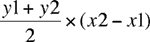Quandaries and Queries Hi. I have a set of XY co-ordinates(say 49 points),I want to find the area enclosed by the curve joining them and the axes.I do not have an equation for the curve and I am not able to fit a curve given the points.I use the trapezoidal rule (Y1+Y2/2)*(X2-X1), Is it right? can I do this with any other method? thank you prashanth Hi Prashanth, The trapezoidal rule is the appropriate method to use, however I don't like the way you have written the expression. Y1+Y2/2 is Y1 plus one half of Y2, but you need one half of the sum of Y2 and Y1. The expresion should bePenny Go to Math Central